## Chemistry Formulas for Atomic Structure

### Chemistry Formulas from Bohr’s Model of Atom

#### ·         Angular momentum of electron in nth orbit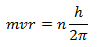Where, m = Mass of the electron,
v = velocity of electron,
r = radius of the orbit,
h = Planck’s constant,
n = no. of orbit in which electron is present,

#### ·         Energy of electron in nth orbit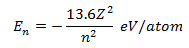Where, Z = Atomic No. of Electron,

#### ·         Energy absorbed or released in an electron jump (E)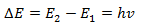#### ·         Radius of orbits of hydrogen like species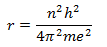For hydrogen atom Z = 1, for first orbit n = 1,
On substituting values of the constants
h = 6.62x10-27erg sec,
m = 9.1x10-28g,
e = 4.8x10-10
we get,
r = 0.529 Å
So, radius of first orbit of hydrogen atom is 0.529 Å.

rn = 0.529n2/Z Å

#### ·         Velocity of electron in nth orbit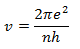On substituting values of the constants
We get,

#### ·         No. of revolution per second made by an electron around the nucleus of atom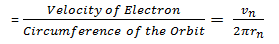#### ·         Energy of electron in nth orbit (En)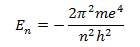On substituting values of the constants
We get,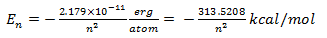In general,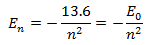#### ·         Energy of electron in a Hydrogen Atom in different energy levels

 Energy Level E (Joules/atom) E (eV/atom) E (kcal/mol) 1 -21.79x10-19 -13.6 -313.5 2 -5.42x10-19 -3.4 -78.4 3 -2.41x10-19 -1.51 -38.84 4 -1.36x10-19 -0.85 -19.6 5 -0.87x10-19 -0.544 -12.5 Infinite 0 0 0

#### ·         Frequency or wave length of emitted radiation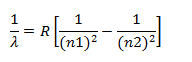Where, λ = wavelength of emitted radiations
R = Rydberg constant for Hydrogen atom

#### ·         Number of spectral lines produced when an electron drops from nth level to ground level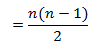### Chemistry Formulas from Photoelectric Effect

E = hv

#### ·         Total energy,

Total Energy = (mv2/2) + w
Where, w = energy required to remove the electron.

### Chemistry Formulas from Wave Mechanical Concept of Atom

#### ·         De Broglie’s Equation,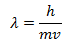Where, m = mass of particle,
v = velocity of the particle,
h = Planck’s Constant,
or,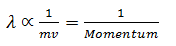### Chemistry Formulas from Heisenberg’s Uncertainty Principle

#### ·        Heisenberg’s Uncertainty Principle,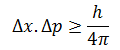Where,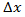= uncertainty in the position of the particle,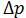= uncertainty in the momentum of the particle,
Also,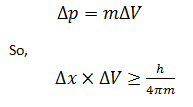### Chemistry Formulas from Quantum Numbers

#### ·         Principle Quantum Number (n),

Maximum no. of electrons in n principle quantum number = 2n2

#### ·         Azimuthal Quantum Number (l),

For the given value of principle quantum number (n), azimuthal quantum number (l), may have all integral values from 0 to (n-1)

#### ·         Magnetic Quantum Number (m),

No. of orbitals in a sub-shell = 2 l +1

#### ·         Spin Quantum Number (s),

For spinning of electron about its own axis

# Chemistry Formulas for Structure of Atom Part 1

## Chemistry Formulas of Structure of Atom

### Chemistry Formulas from Rutherford Atomic Model

·         Radius of Nucleus, rn = r0 × A1/3
Where, A = Mass Number,
r0 = Proportionality Constant = 1.4 × 10-13 cm
·         Volume of the nucleus = Approx. 10-39 cm3
·         Volume of the atom = Approx. 10-24 cm3
·         Density of the nucleus = 1014 g cm-3
Or,
Important Characteristics of Three Fundamental Particles
1.      Electron
·         Charge on an Electron = -1.602×10-19 coulombs.
·         Mass of an Electron = 9.11×10-28 g
·         Specific Charge (e/m ratio) of electrons (cathode rays) = 1.76×108 coulombs/gram
·         Radius of the electron = 10-15 cm
·         Density of the electron = 2.17×1017g/cc
·         Mass of one mole of the electrons = Approx. 0.55mg
·         Charge on one mole of the electrons = 96500 Coulombs = 1 Faraday
2.      Proton
·         Mass of Proton = 1.672×10-24g
·         Charge on Proton = 1.602×10-19 Coulombs
·         Specific Charge of Proton = 9.58×104 Coulombs/gram
·         Mass of one mole of proton = 1.007 gram
·         Charge on one mole of proton = 96500 Coulombs = 1 Faraday
·         Volume of Proton = Approx. 1.5×10-38cm3
3.      Neutron
·         Mass of Neutron = 1.675×10-24g
·         Specific Charge on Neutron = 0
·         Density of Neutron = 1.5×1014g/cc
·         Mass of one mole of neutron = 1.008g
4.      Other Sub-Atomic Particles of Atom
·         Positrons
·         Neutrions
·         Mesons
Chemistry Formulas of Atomic Number (Z) and Mass Number (A)
·         General Symbol for an Atom of Element (E) indicating its Atomic Number (Z) and Mass Number (A)
ZEA
·         Atomic Number (Z) = Number of Protons = Number of Electrons
·         Mass Number (A) = Number of Protons + Number of Neutrons
·         No. of Neutrons = A – Z

# Chemistry Formula for Atomic Structure Part 2

## Chemistry formulas for Atoms, Molecules and Chemical Arithmetic Part II

### Gram Atomic Weight (GAW) related Chemical Formulas

·         No. of Gram Atoms or Mole Atoms = Weight of an Element/GAW
·         Weight of an Element in gram = No. of Gram Atoms x GAW
·         No. of atoms in 1 GAW = 6.02x1023
·         No. of atoms in given substance = 6.02x1023 x Weight/GAW
·         No. of atoms in 1 gram of an element = 6.02x1023/Atomic Weight

### Gram Molecular Weight (GMW) related Chemical Formulas

·         No. of Gram molecules or Mole Molecules = Weight of Substance/GMW
·         Weight of Substance in gram = No. of Gram Molecules x GMW
·         Avogadro’s No. = 6.02x1023 per mol

### Mole Concept related Chemical Formulas

·         1 mole contains 6.02x1023 particles
·         1 mole of an atom = 1 GAW of it
·         1 mole of a compound = 1 GMW of it
·         Examples, 1 mole of Na = 23 g
·         1 mole of H2O = 18 g
·         1 mole of OH- ions = 17 g
·         1 mole = 1 gram molecules
·         1 mole = 1 gram molecular weight
·         1 mole = 22.4 litres at NTP
·         1 mole = 6.02x1023 molecules
·         1 mole = 1 gram atomic weight
·         1 mole = 6.02x1023 atoms
·         No. of Moles = Weight of Substance in Gram/Gram Molecular Weight
·         No. of Moles = No. of Unitary Particles/Avogadro’s No.
·         No. of Moles = Volume in Litres at NTP/22.4 Litres

### Gram Molecular Volume related Chemical Formulas

·         1 Gram Molar Volume = 22.4 Litres
·         Example, Volume of 16 gram (1 mole) of CH4 at STP= 22.4 Litres
·         Volume of 2 gram (1 mole) of H2 at STP = 22.4 Litres
·         Weight of 11.2 litres of any Gas at STP = VD (Vapour Density) of that Gas in Gram
·         Density of Gas at NTP = Molar Weight in Gram/22400 mL

## Atomic Weight related Chemical Formulas

·         Atomic Weight of an Element = Weight of an average Atom of that Element/ (1/12)x Mass of an element of C12
·         1 a.m.u. = 1.66x10-24g
·         Atomic Weight = Gram Atomic Weight (GAW)
·          1 Gram Atomic Weight (GAW) of every element contains 6.023x1023 atoms of that element.
·         No. of gram of an element = weight of element in gram/ Gram Atomic Weight (GAW) of that element

### Methods of Determining Atomic Weight

#### i.     Dulong and Pettits Method:

Applicable only for solid elements except Be, B, C, Si.
·      Atomic Weight  x Specific Heat = 6.4 (app.)
·      Atomic Weight  (app.) = 6.4/ Specific Heat (in Calories)
·      Exact Atomic Weight = Equivalent Weight x Valency
·      Valency = App. Atomic Weight / Equivalent Weight

#### ii.     Vapour Density Method:

Applicable only for those elements whose chlorides are volatile.
·         Valency of the Element = Molecular Weight of Chloride / Equivalent Weight of Chloride
·         Valency of the Element = (2 x V.D. of Chloride) / (Equivalent Weight of Metal + 35.5)
Where, V.D. = Vapour Density
·         Atomic Weight = Equivalent Weight of Metal x Valency

#### iii.     Specific Heat Method:

Applicable only for Gases.
·         Cp/Cv for monoatomic gases = 1.66
·         Cp/Cv for diatomic gases = 1.40
·         Cp/Cv for triatomic gases = 1.33
·         Atomic Weight of Gaseous Element = Molecular Weight/ Atomicity
Where, Atomicity is number of atoms present in a molecule of a gaseous element. For example atomicity of Inert Gas is 1, atomicity of Ozone is 3, atomicity of H2 N2 O2 X2 is 2, and atomicity of Sulphur is 8.

#### iv.     Volatile Chloride Formation Method:

·         Atomic Weight of the Element = Equivalent Weight (Z) x Valency (x)

#### v.     Isomorphism Method:

This method based on law of Isomorphism. According to law of Isomorphism, “Compounds having identical crystal structure have similar constitution and chemical formula”
·         Atomic Weight = Equivalent Weight x Valency
·         Weight of Element A that combines with certain weight of other elements/Weight of Element B that combines with the same weight of other elements = Atomic Weight of A / Atomic Weight of B

## Molecular Weight related Chemical Formulas

·         Molecular Weight = Weight of 1 Molecule of the Substance/ (1/12)x Weight of 1 atom of C12
·         Actual Weight of 1 Molecule = Molecular Weight x 1.66x10-24g

### Methods of Determining Atomic Weight

#### 1.              Diffusion Method:

Applicable only for gases.
·

Where,  r1 & r2 is rate of diffusion of gases and M1 & M2 is Molecular Weight.

#### 2.              Vapour Density Method:

Applicable only for gases.
·         Molecular Weight = 2 x Vapour Density

#### 3.              Victor Mayer Method:

Applicable only for volatile liquids and solids.
·         Molecular Weight of a substance = 22400 ml of vapour of a substance at STP

## Equivalent Weight related Chemical Formulas

·         No. of Gram Equivalent Weight = Weight of the substance in gram/ Gram Equivalent Weight of the substance
·         Equivalent Weight of an Element = Atomic Weight/ Valency
·         Equivalent Weight of an Acid = Molecular Weight/ Basicity
·         Equivalent Weight of an Base = Molecular Weight/ Acidity
·         Equivalent Weight of a Salt = Formula Weight/ Total Positive or Negative Charge
·         Equivalent Weight of a Reducing Agent = Formula Weight/ No. of electrons lost per molecule or Total change in Oxidation Number
·         Equivalent Weight of an Oxidising Agent = Formula Weight/ No. of electrons gained per molecule or Total change in Oxidation Number
·         Equivalent Weight of Radicals = Formula Weight of Radical/ No. of units of Charge

### Methods of Determining Equivalent Weight

#### 1.              Hydrogen Displacement Method:

Applicable for metals which can displace or combine with hydrogen.
·         Equivalent Weight of Metal = (Weight of metal x 1.008)/ Weight of Hydrogen Displaced
·         Equivalent Weight of Metal = (Weight of metal x 11200)/ Volume in ml of H2 displaced at STP

#### 2.              Oxide Formation Method:

·         Equivalent Weight of Metal = (Weight of metal x 8)/ Weight of Oxygen
·         Equivalent Weight of Metal = (Weight of metal x 5600)/ Volume in ml of Oxygen at STP

#### 3.              Chloride Formation Method:

·         Equivalent Weight of Metal = (Weight of metal x 35.5)/ Weight of Chlorine
·         Equivalent Weight of Metal = (Weight of metal x 11200)/ Volume in ml of Chlorine at STP

#### 4.              Neutralization Method:

·         Equivalent Weight of Acid or Base = Weight of  acid or base in gram/ (Volume of base or acid in litre required for neutralization x Normality of base or acid)

#### 5.              Metal Displacement Method:

·         Weight of Metal Added W1/ Weight of Metal Displaced W2 = Equivalent Weight of Metal Added E1/ Equivalent Weight of Metal Displaced E2

#### 6.              Electrolytic Method:

·         Gram Equivalent Weight = Electrochemical Equivalent x 96500
·         Weight of X deposited/ Weight of Y deposited = Equivalent Weight of X/ Equivalent Weight of Y

#### 7.              Double Decomposition Method:

·         Weight of Salt taken (W1)/ Weight of ppt. obtained (W2) = Equivalent Weight of Salt (E1)/ Equivalent Weight of Salt in ppt. (E2)

#### 8.              Conversion Method:

·         Weight of Compound A (W1)/ Weight of Compound B (W2) = (Equivalent Weight of Metal + Equivalent Weight of Anion of Compound A)/ (Equivalent Weight of Metal + Equivalent Weight of Anion of Compound B)

#### 9.              Volatile Chloride Method:

·         Equivalent Weight = {(2 x Vapour Density of Chloride)/ Valency} – 35.5

#### 10.          Silver Salt Method:

Applicable for organic acids
·         Equivalent Weight of Acid = Molecular Weight of Acid/ Basicity## NOTE:

If error occur=> Change website url from www.chemistrynotesinfo.blogspot.in to www.chemistrynotesinfo.com